Скачать презентацию A progress report on using Maude to verify

47d23c826007c3c2f56247f880278785.ppt

• Количество слайдов: 22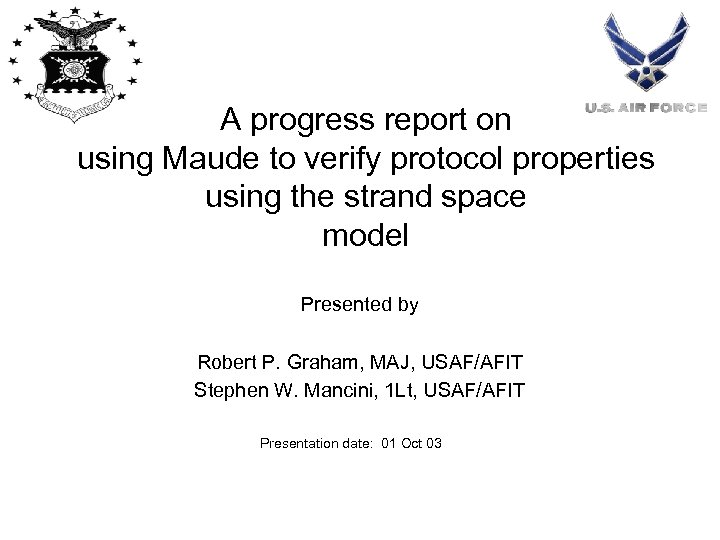A progress report on using Maude to verify protocol properties using the strand space model Presented by Robert P. Graham, MAJ, USAF/AFIT Stephen W. Mancini, 1 Lt, USAF/AFIT Presentation date: 01 Oct 03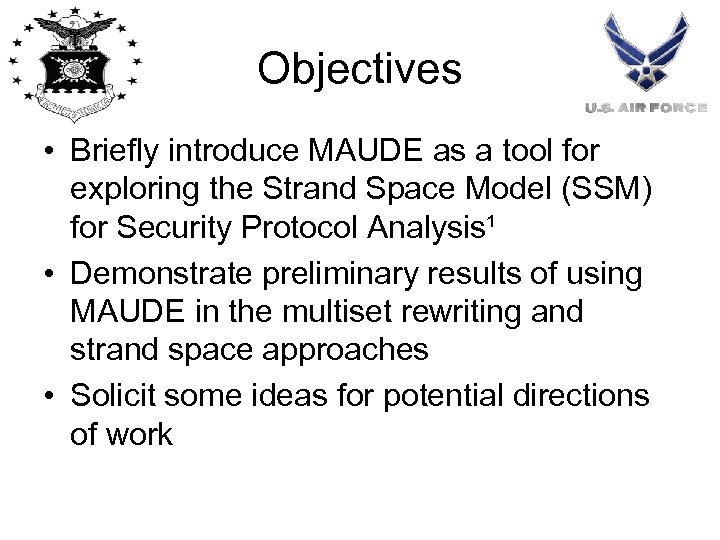Objectives • Briefly introduce MAUDE as a tool for exploring the Strand Space Model (SSM) for Security Protocol Analysis¹ • Demonstrate preliminary results of using MAUDE in the multiset rewriting and strand space approaches • Solicit some ideas for potential directions of work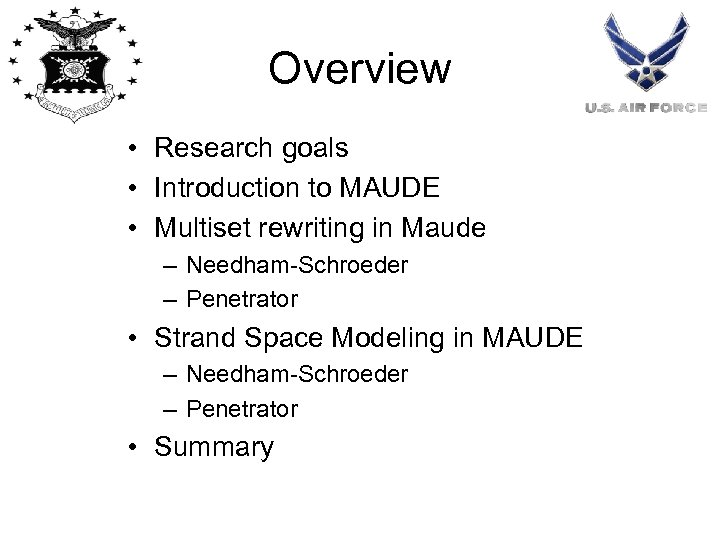Overview • Research goals • Introduction to MAUDE • Multiset rewriting in Maude – Needham-Schroeder – Penetrator • Strand Space Modeling in MAUDE – Needham-Schroeder – Penetrator • Summary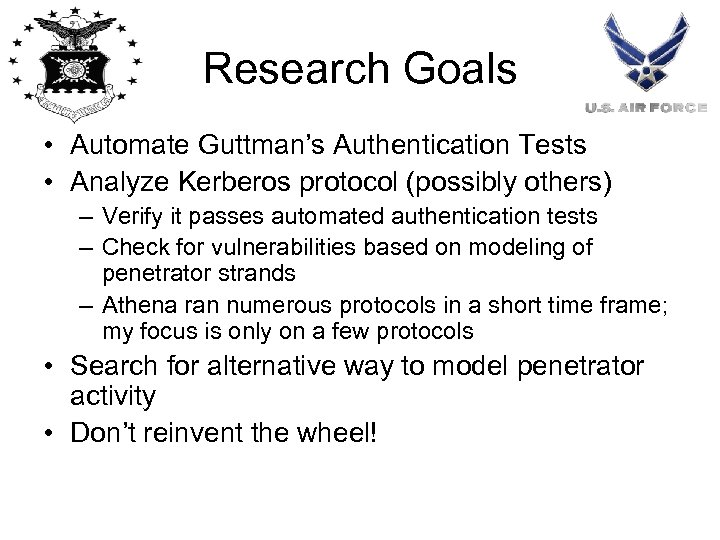Research Goals • Automate Guttman’s Authentication Tests • Analyze Kerberos protocol (possibly others) – Verify it passes automated authentication tests – Check for vulnerabilities based on modeling of penetrator strands – Athena ran numerous protocols in a short time frame; my focus is only on a few protocols • Search for alternative way to model penetrator activity • Don’t reinvent the wheel!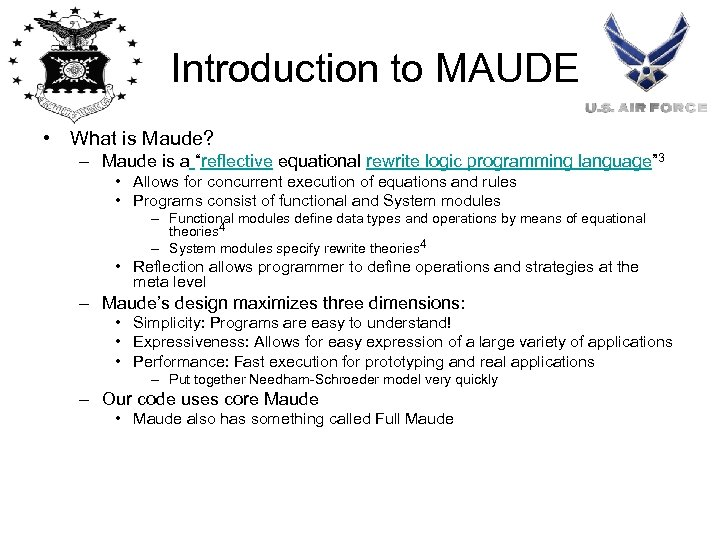Introduction to MAUDE • What is Maude? – Maude is a “reflective equational rewrite logic programming language” 3 • Allows for concurrent execution of equations and rules • Programs consist of functional and System modules – Functional modules define data types and operations by means of equational theories 4 – System modules specify rewrite theories 4 • Reflection allows programmer to define operations and strategies at the meta level – Maude’s design maximizes three dimensions: • Simplicity: Programs are easy to understand! • Expressiveness: Allows for easy expression of a large variety of applications • Performance: Fast execution for prototyping and real applications – Put together Needham-Schroeder model very quickly – Our code uses core Maude • Maude also has something called Full Maude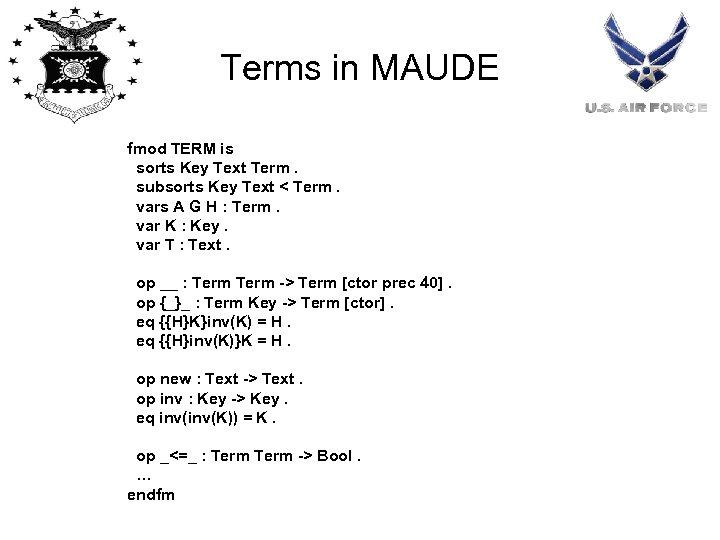Terms in MAUDE fmod TERM is sorts Key Text Term. subsorts Key Text < Term. vars A G H : Term. var K : Key. var T : Text. op __ : Term -> Term [ctor prec 40]. op {_}_ : Term Key -> Term [ctor]. eq {{H}K}inv(K) = H. eq {{H}inv(K)}K = H. op new : Text -> Text. op inv : Key -> Key. eq inv(K)) = K. op _<=_ : Term -> Bool. … endfm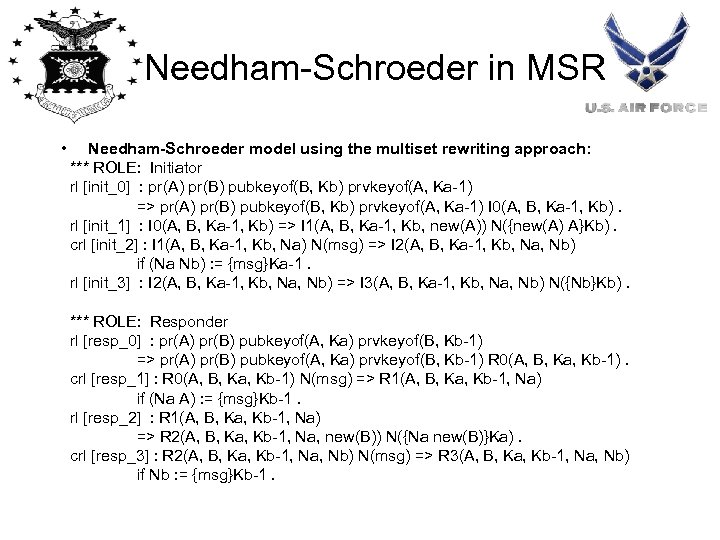Needham-Schroeder in MSR • Needham-Schroeder model using the multiset rewriting approach: *** ROLE: Initiator rl [init_0] : pr(A) pr(B) pubkeyof(B, Kb) prvkeyof(A, Ka-1) => pr(A) pr(B) pubkeyof(B, Kb) prvkeyof(A, Ka-1) I 0(A, B, Ka-1, Kb). rl [init_1] : I 0(A, B, Ka-1, Kb) => I 1(A, B, Ka-1, Kb, new(A)) N({new(A) A}Kb). crl [init_2] : I 1(A, B, Ka-1, Kb, Na) N(msg) => I 2(A, B, Ka-1, Kb, Na, Nb) if (Na Nb) : = {msg}Ka-1. rl [init_3] : I 2(A, B, Ka-1, Kb, Na, Nb) => I 3(A, B, Ka-1, Kb, Na, Nb) N({Nb}Kb). *** ROLE: Responder rl [resp_0] : pr(A) pr(B) pubkeyof(A, Ka) prvkeyof(B, Kb-1) => pr(A) pr(B) pubkeyof(A, Ka) prvkeyof(B, Kb-1) R 0(A, B, Ka, Kb-1). crl [resp_1] : R 0(A, B, Ka, Kb-1) N(msg) => R 1(A, B, Ka, Kb-1, Na) if (Na A) : = {msg}Kb-1. rl [resp_2] : R 1(A, B, Ka, Kb-1, Na) => R 2(A, B, Ka, Kb-1, Na, new(B)) N({Na new(B)}Ka). crl [resp_3] : R 2(A, B, Ka, Kb-1, Na, Nb) N(msg) => R 3(A, B, Ka, Kb-1, Na, Nb) if Nb : = {msg}Kb-1.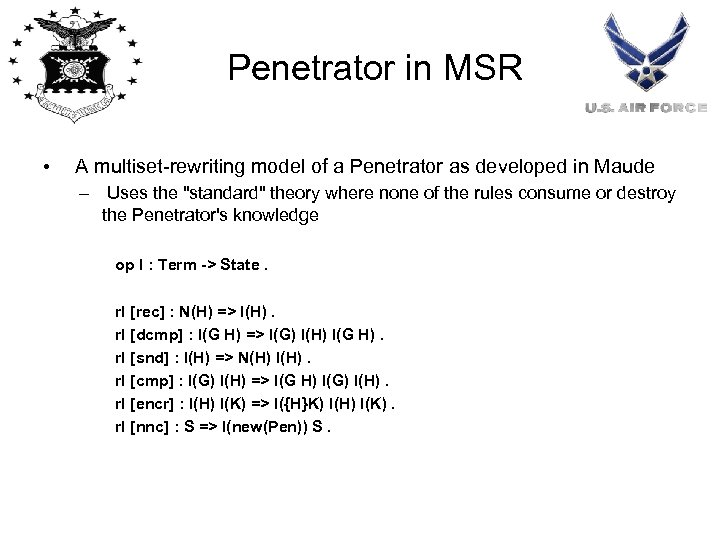Penetrator in MSR • A multiset-rewriting model of a Penetrator as developed in Maude – Uses the "standard" theory where none of the rules consume or destroy the Penetrator's knowledge op I : Term -> State. rl [rec] : N(H) => I(H). rl [dcmp] : I(G H) => I(G) I(H) I(G H). rl [snd] : I(H) => N(H) I(H). rl [cmp] : I(G) I(H) => I(G H) I(G) I(H). rl [encr] : I(H) I(K) => I({H}K) I(H) I(K). rl [nnc] : S => I(new(Pen)) S.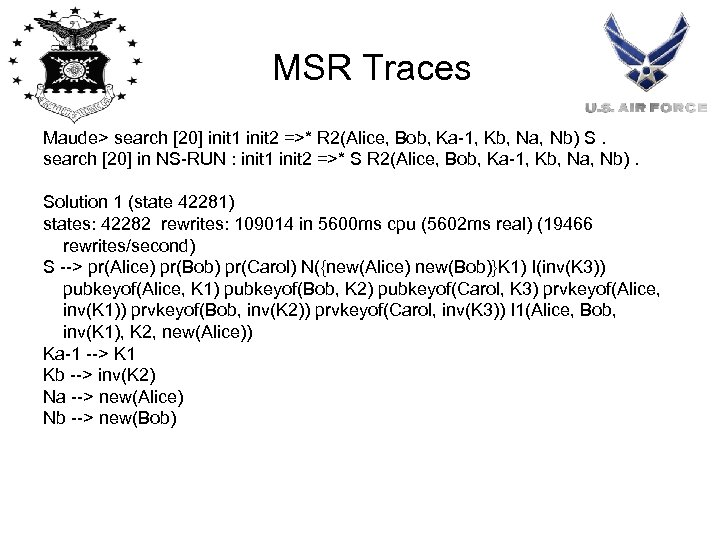MSR Traces Maude> search  init 1 init 2 =>* R 2(Alice, Bob, Ka-1, Kb, Na, Nb) S. search  in NS-RUN : init 1 init 2 =>* S R 2(Alice, Bob, Ka-1, Kb, Na, Nb). Solution 1 (state 42281) states: 42282 rewrites: 109014 in 5600 ms cpu (5602 ms real) (19466 rewrites/second) S --> pr(Alice) pr(Bob) pr(Carol) N({new(Alice) new(Bob)}K 1) I(inv(K 3)) pubkeyof(Alice, K 1) pubkeyof(Bob, K 2) pubkeyof(Carol, K 3) prvkeyof(Alice, inv(K 1)) prvkeyof(Bob, inv(K 2)) prvkeyof(Carol, inv(K 3)) I 1(Alice, Bob, inv(K 1), K 2, new(Alice)) Ka-1 --> K 1 Kb --> inv(K 2) Na --> new(Alice) Nb --> new(Bob)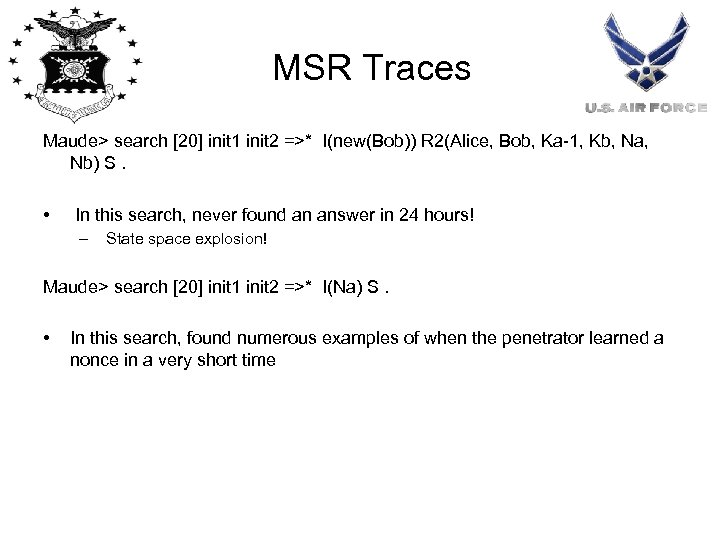MSR Traces Maude> search  init 1 init 2 =>* I(new(Bob)) R 2(Alice, Bob, Ka-1, Kb, Na, Nb) S. • In this search, never found an answer in 24 hours! – State space explosion! Maude> search  init 1 init 2 =>* I(Na) S. • In this search, found numerous examples of when the penetrator learned a nonce in a very short timeMSR Lessons Learned • Maude suffered from state space explosion • Did well when searching for individual states • Good learning tool!Strand Spaces in MAUDE • A single rewrite rule “executes” a set of strands: var S T : Strand. var H : Term. rl [label-p-reduction] : + H S | - H T => S | T.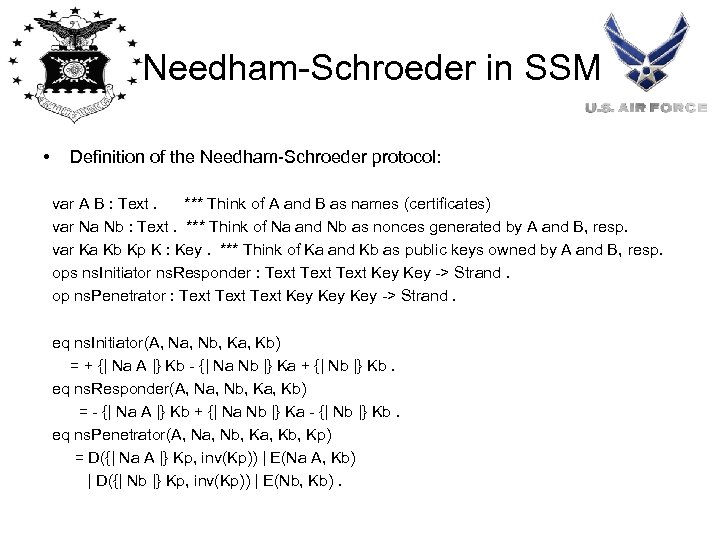Needham-Schroeder in SSM • Definition of the Needham-Schroeder protocol: var A B : Text. *** Think of A and B as names (certificates) var Na Nb : Text. *** Think of Na and Nb as nonces generated by A and B, resp. var Ka Kb Kp K : Key. *** Think of Ka and Kb as public keys owned by A and B, resp. ops ns. Initiator ns. Responder : Text Key -> Strand. op ns. Penetrator : Text Key Key -> Strand. eq ns. Initiator(A, Na, Nb, Ka, Kb) = + {| Na A |} Kb - {| Na Nb |} Ka + {| Nb |} Kb. eq ns. Responder(A, Na, Nb, Ka, Kb) = - {| Na A |} Kb + {| Na Nb |} Ka - {| Nb |} Kb. eq ns. Penetrator(A, Na, Nb, Ka, Kb, Kp) = D({| Na A |} Kp, inv(Kp)) | E(Na A, Kb) | D({| Nb |} Kp, inv(Kp)) | E(Nb, Kb).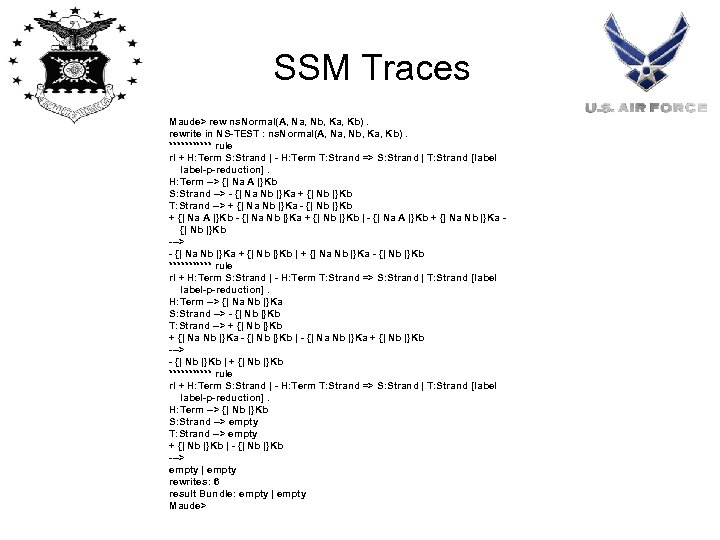SSM Traces Maude> rew ns. Normal(A, Na, Nb, Ka, Kb). rewrite in NS-TEST : ns. Normal(A, Na, Nb, Ka, Kb). ****** rule rl + H: Term S: Strand | - H: Term T: Strand => S: Strand | T: Strand [label-p-reduction]. H: Term --> {| Na A |}Kb S: Strand --> - {| Na Nb |}Ka + {| Nb |}Kb T: Strand --> + {| Na Nb |}Ka - {| Nb |}Kb + {| Na A |}Kb - {| Na Nb |}Ka + {| Nb |}Kb | - {| Na A |}Kb + {| Na Nb |}Ka {| Nb |}Kb ---> - {| Na Nb |}Ka + {| Nb |}Kb | + {| Na Nb |}Ka - {| Nb |}Kb ****** rule rl + H: Term S: Strand | - H: Term T: Strand => S: Strand | T: Strand [label-p-reduction]. H: Term --> {| Na Nb |}Ka S: Strand --> - {| Nb |}Kb T: Strand --> + {| Nb |}Kb + {| Na Nb |}Ka - {| Nb |}Kb | - {| Na Nb |}Ka + {| Nb |}Kb ---> - {| Nb |}Kb | + {| Nb |}Kb ****** rule rl + H: Term S: Strand | - H: Term T: Strand => S: Strand | T: Strand [label-p-reduction]. H: Term --> {| Nb |}Kb S: Strand --> empty T: Strand --> empty + {| Nb |}Kb | - {| Nb |}Kb ---> empty | empty rewrites: 6 result Bundle: empty | empty Maude>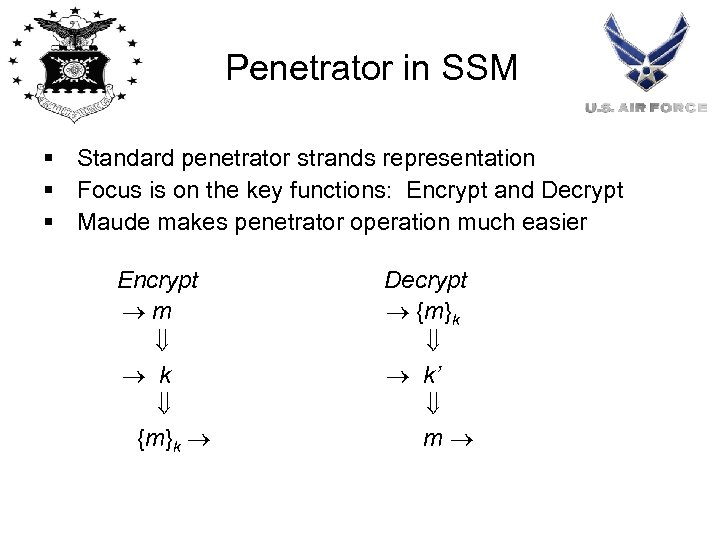Penetrator in SSM § Standard penetrator strands representation § Focus is on the key functions: Encrypt and Decrypt § Maude makes penetrator operation much easier Encrypt m k {m}k Decrypt {m}k k’ m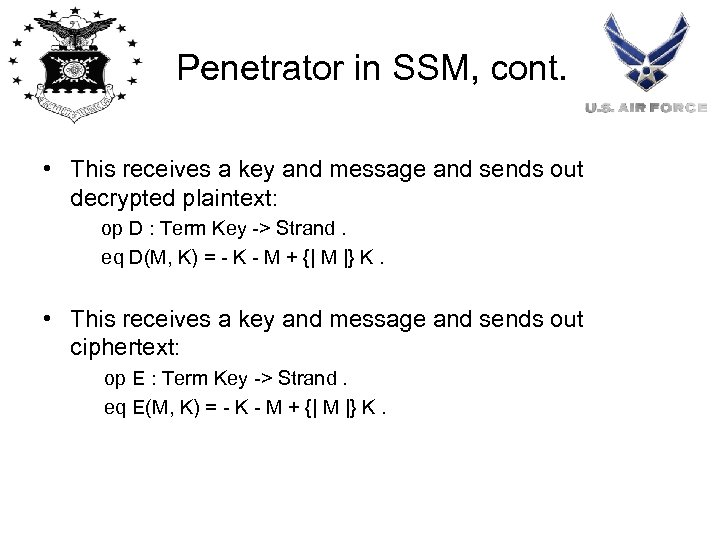Penetrator in SSM, cont. • This receives a key and message and sends out decrypted plaintext: op D : Term Key -> Strand. eq D(M, K) = - K - M + {| M |} K. • This receives a key and message and sends out ciphertext: op E : Term Key -> Strand. eq E(M, K) = - K - M + {| M |} K.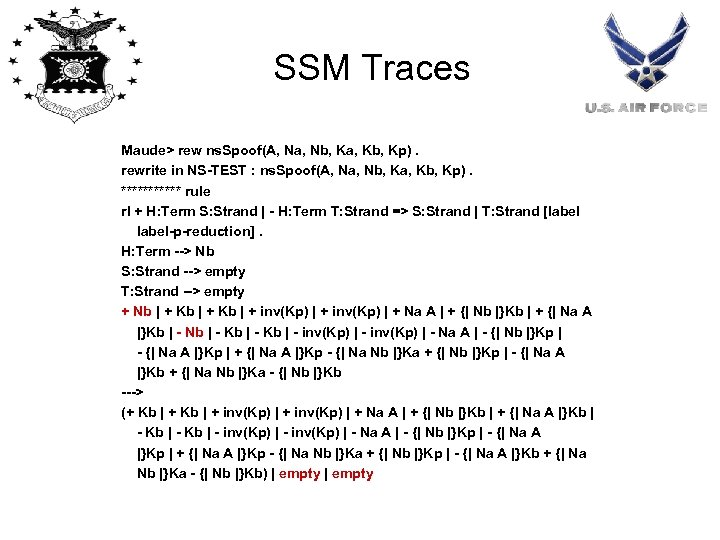SSM Traces Maude> rew ns. Spoof(A, Na, Nb, Ka, Kb, Kp). rewrite in NS-TEST : ns. Spoof(A, Na, Nb, Ka, Kb, Kp). ****** rule rl + H: Term S: Strand | - H: Term T: Strand => S: Strand | T: Strand [label-p-reduction]. H: Term --> Nb S: Strand --> empty T: Strand --> empty + Nb | + Kb | + inv(Kp) | + Na A | + {| Nb |}Kb | + {| Na A |}Kb | - Nb | - Kb | - inv(Kp) | - Na A | - {| Nb |}Kp | - {| Na A |}Kp | + {| Na A |}Kp - {| Na Nb |}Ka + {| Nb |}Kp | - {| Na A |}Kb + {| Na Nb |}Ka - {| Nb |}Kb ---> (+ Kb | + inv(Kp) | + Na A | + {| Nb |}Kb | + {| Na A |}Kb | - inv(Kp) | - Na A | - {| Nb |}Kp | - {| Na A |}Kp | + {| Na A |}Kp - {| Na Nb |}Ka + {| Nb |}Kp | - {| Na A |}Kb + {| Na Nb |}Ka - {| Nb |}Kb) | empty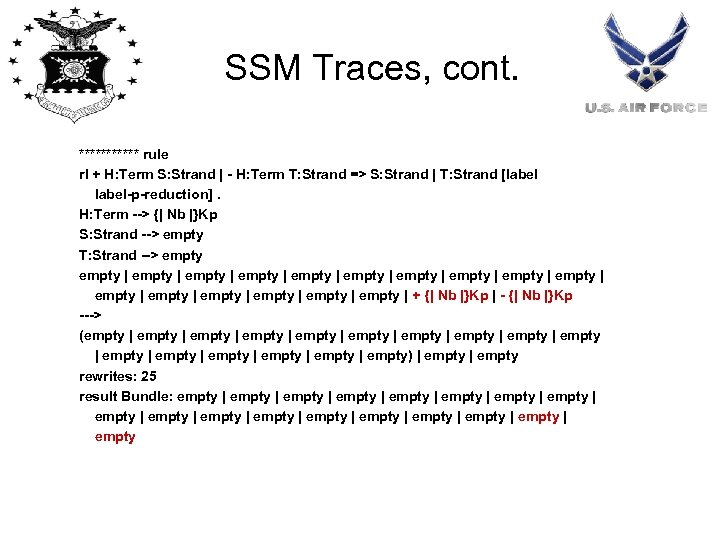SSM Traces, cont. ****** rule rl + H: Term S: Strand | - H: Term T: Strand => S: Strand | T: Strand [label-p-reduction]. H: Term --> {| Nb |}Kp S: Strand --> empty T: Strand --> empty | empty | empty | empty | empty | + {| Nb |}Kp | - {| Nb |}Kp ---> (empty | empty | empty | empty | empty) | empty rewrites: 25 result Bundle: empty | empty | empty | empty | empty | empty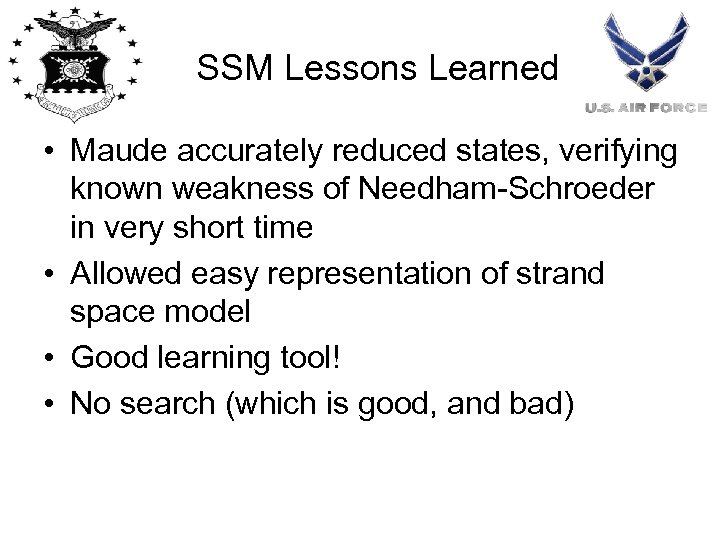SSM Lessons Learned • Maude accurately reduced states, verifying known weakness of Needham-Schroeder in very short time • Allowed easy representation of strand space model • Good learning tool! • No search (which is good, and bad)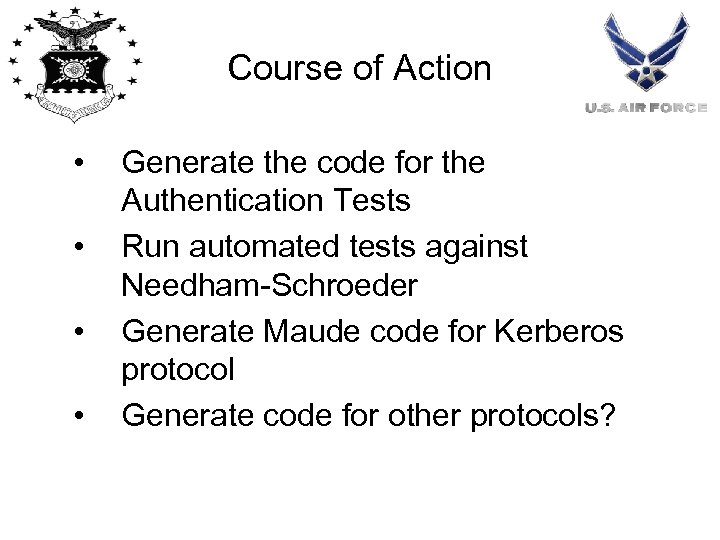Course of Action • • Generate the code for the Authentication Tests Run automated tests against Needham-Schroeder Generate Maude code for Kerberos protocol Generate code for other protocols?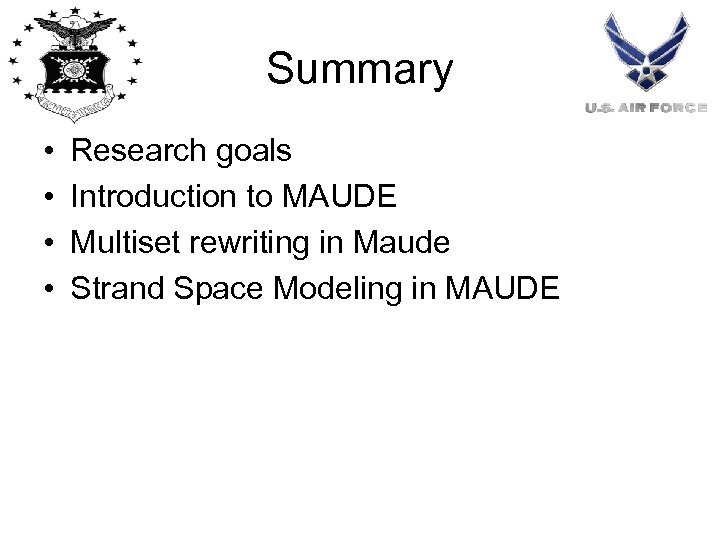Summary • • Research goals Introduction to MAUDE Multiset rewriting in Maude Strand Space Modeling in MAUDE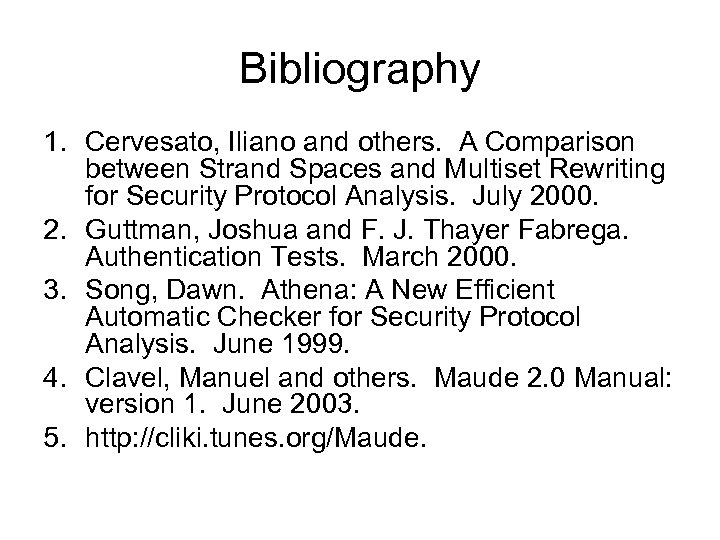Bibliography 1. Cervesato, Iliano and others. A Comparison between Strand Spaces and Multiset Rewriting for Security Protocol Analysis. July 2000. 2. Guttman, Joshua and F. J. Thayer Fabrega. Authentication Tests. March 2000. 3. Song, Dawn. Athena: A New Efficient Automatic Checker for Security Protocol Analysis. June 1999. 4. Clavel, Manuel and others. Maude 2. 0 Manual: version 1. June 2003. 5. http: //cliki. tunes. org/Maude.